# 7. -14 points SerCP1116.6.P.037. My Notes A Teat. DR Given a 1.50 pF capacitor, a 6.00...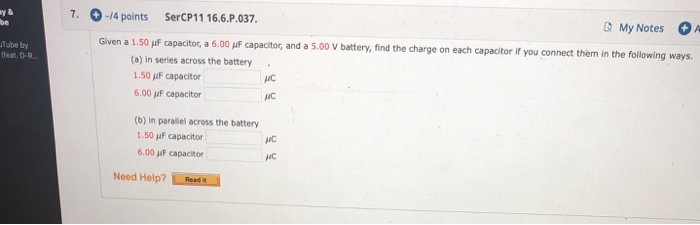7. -14 points SerCP1116.6.P.037. My Notes A Teat. DR Given a 1.50 pF capacitor, a 6.00 uF capacitor, and a 5.00 V battery, find the charge on each capacitor if you connect them in the following ways. (a) in series across the battery 1.50 pF capacitor 6.00 F capacitor (b) in parallel across the battery 1.50 f capacitor 6.00 iF capacitor Need Help?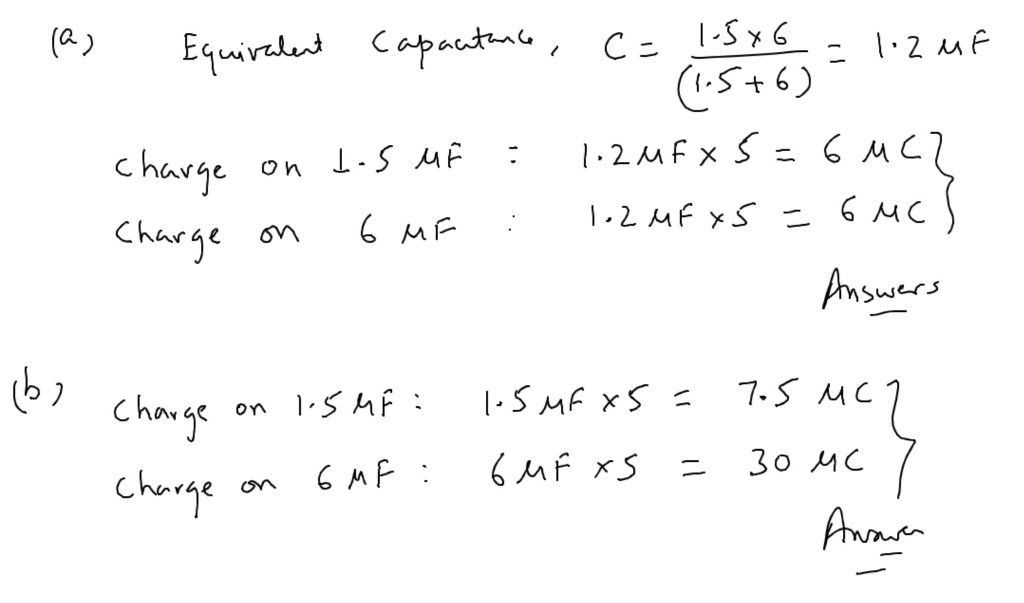#### Earn Coin

Coins can be redeemed for fabulous gifts.

Similar Homework Help Questions
• ### 10. 14 points SerCP11 16.P037 My Notes Ask Your Teac Given a 2.75 μF capacitor, a...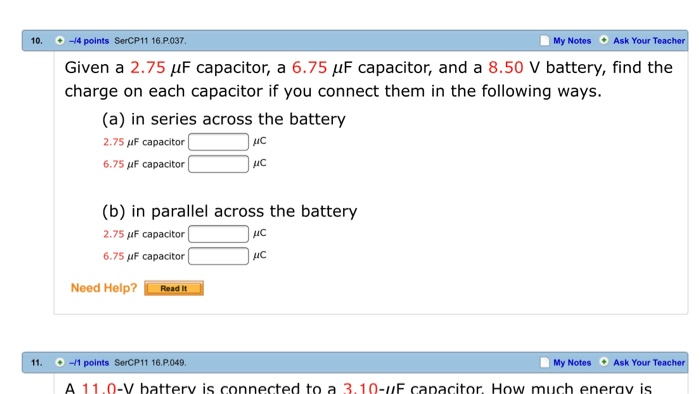10. 14 points SerCP11 16.P037 My Notes Ask Your Teac Given a 2.75 μF capacitor, a 6.75μF capacitor, and a 8.50 V battery, find the charge on each capacitor if you connect them in the following ways. (a) in series across the battery 2.75 μF capacitor 6.75 uF capacitor AIC (b) in parallel across the battery 2.75 uF capacitor 6.75 μF capacitor AC Need Help? Read It 11. 1 points SerCP11 16.P049 My Notes Ask Your Teac A 11.0-V battery...

• ### Given a 2.75 µF capacitor, a 6.00 µF capacitor, and a 9.00 V battery, find the...

Given a 2.75 µF capacitor, a 6.00 µF capacitor, and a 9.00 V battery, find the charge on each capacitor if you connect them in the following ways.(a) in series across the batteryµC(b) in parallel across the battery2.75 µF capacitor µC6.00 µF capacitor µC

• ### If both questions can be answered thank you :) 4. (-/1.25 Points] DETAILS MY NOTES ASK...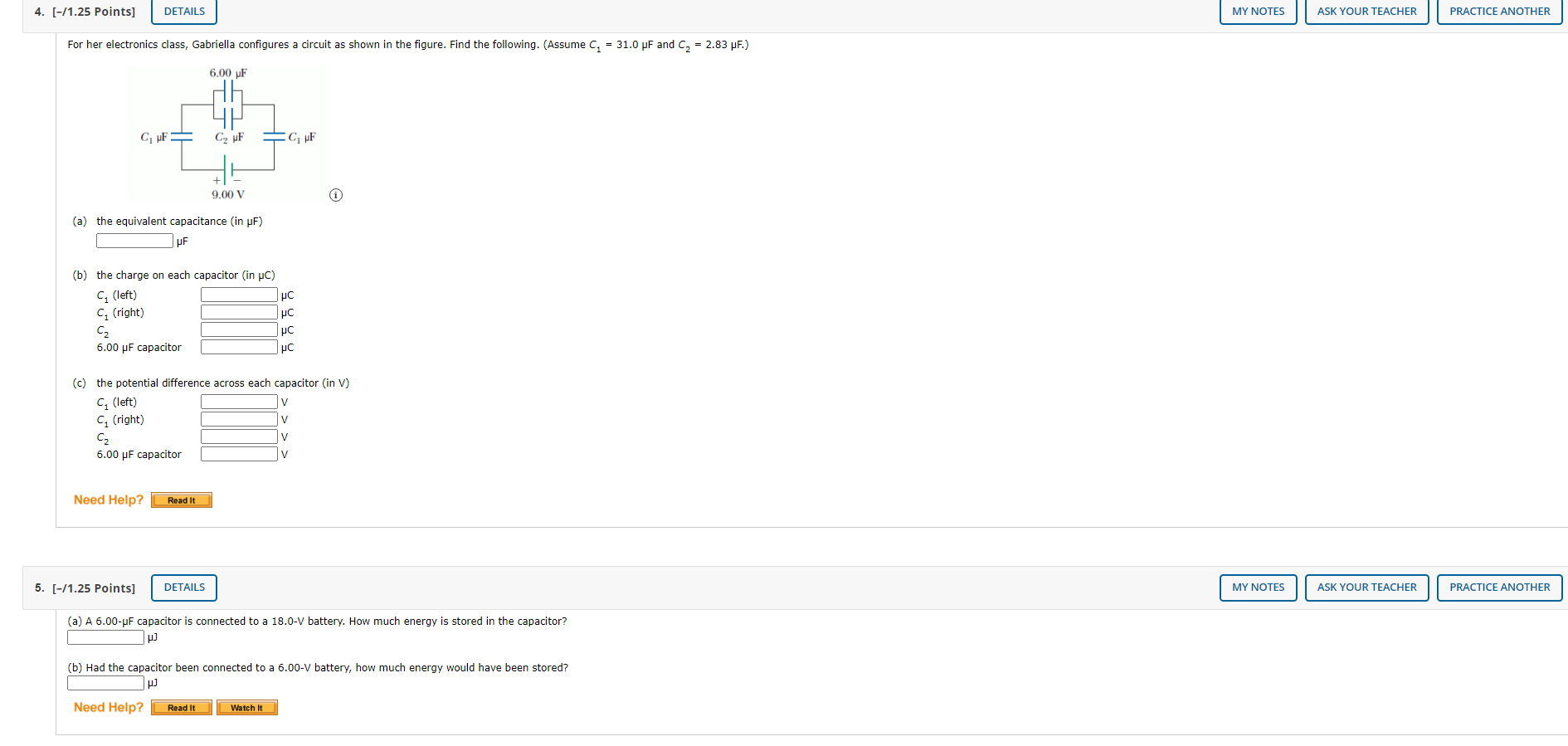If both questions can be answered thank you :) 4. (-/1.25 Points] DETAILS MY NOTES ASK YOUR TEACHER PRACTICE ANOTHER For her electronics class, Gabriella configures a circuit as shown in the figure. Find the following. (Assume C2 = 31.0 pF and C2 = 2.83 pF.) 6.00 F GF C, F CF 9.00 V (a) the equivalent capacitance (in pF) (b) the charge on each capacitor (in PC) C (left) PC Грс Tμο 6.00 uF capacitor Грс (right) (left) (c)...

• ### 7 4-/1 points SerPSE10 25.3.0P.008. My Notes Ask Your Teacher Two capacitors (C, 2.00 pF and...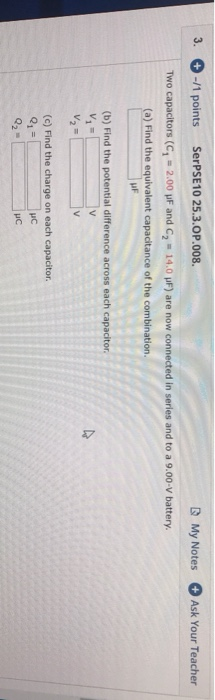7 4-/1 points SerPSE10 25.3.0P.008. My Notes Ask Your Teacher Two capacitors (C, 2.00 pF and C, 14.0 uF) are now connected in series and to a 9.00-V battery. (a) Find the equivalent capacitance of the combination. (b) Find the potential difference across each capacitor V= V V (c) Find the charge on each capacitor. 1 2

• ### 7. -12 points My Notes Ask Your Teacher A 13.7 V battery is connected across points...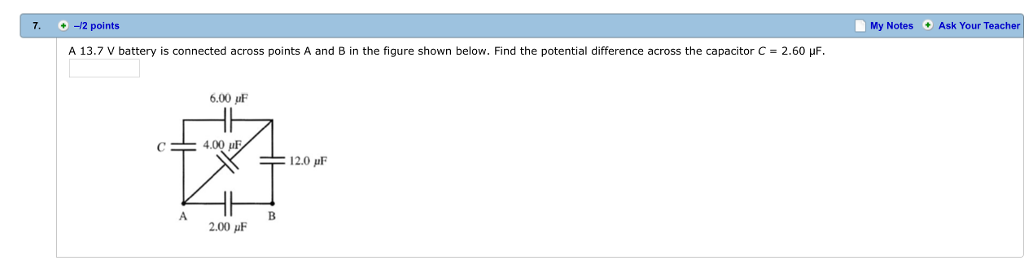7. -12 points My Notes Ask Your Teacher A 13.7 V battery is connected across points A and B in the figure shown below. Find the potential difference across the capacitor C = 2.60 μF 6.00 pF 4.00 uF 12.0 μF 2.00 μF

• ### Given a 1.75 μF capacitor, a 3.25 μF capacitor, and a 4.00 V battery, find the...

Given a 1.75 μF capacitor, a 3.25 μF capacitor, and a 4.00 V battery, find the charge on each capacitor if you connect them in the following ways. (a) in series across the battery 1.75 μF capacitor μC 3.25 μF capacitor μC (b) in parallel across the battery 1.75 μF capacitor μC 3.25 μF capacitor μC

• ### The circuit in the figure below contains a 90.0 V battery and four capacitors. In the...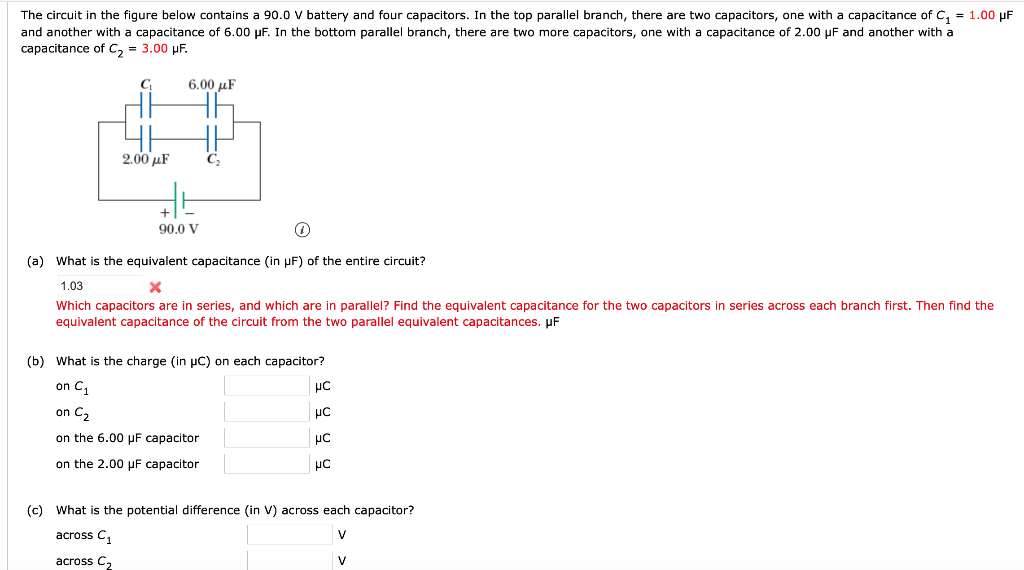The circuit in the figure below contains a 90.0 V battery and four capacitors. In the top parallel branch, there are two capacitors, one with a capacitance of C = 1.00 pF and another with a capacitance of 6.00 pF. In the bottom parallel branch, there are two more capacitors, one with a capacitance of 2.00 pF and another with a capacitance of C2 = 3.00 uF. C 6.00 uF 2.00 uF 90.0 V (a) What is the equivalent capacitance...

• ### 2. -/1 points SerPSE10 25.3.OP.007. My Notes Ask Your Teache Two capacitors, C6.00 pF and C,...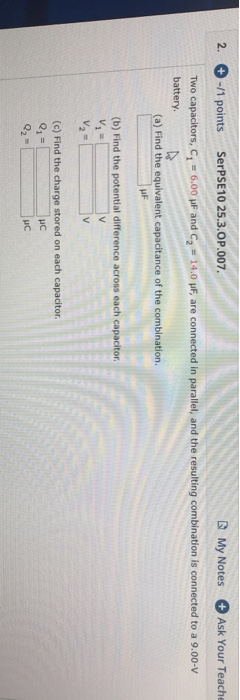2. -/1 points SerPSE10 25.3.OP.007. My Notes Ask Your Teache Two capacitors, C6.00 pF and C, battery 14.00 pF, are connected in parallel, and the resulting combination is connected to a 9.00-V (a) Find the equivalent capacitance of the combination. (b) Find the potential difference across each capacitor V V2= V (c) Find the charge stored on each capacitor. 1= Q2=

• ### My Notes O Ask Your Teacher 0-6.66 points KatzPSEI 29 p 059 | 14. and emf...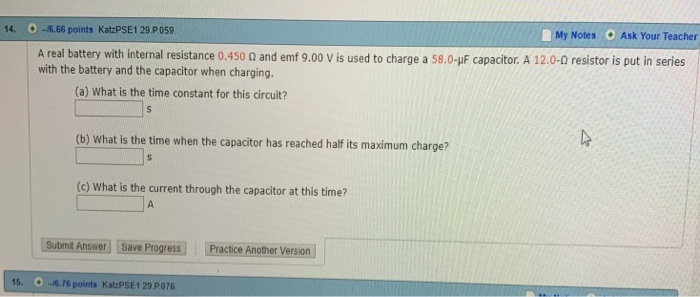My Notes O Ask Your Teacher 0-6.66 points KatzPSEI 29 p 059 | 14. and emf 9.00 V is used to charge a 58.0-uF capacitor. A 12.0-0 resistor is put in series A real battery with internal resistance 0.450 with the battery and the capacitor when charging. (a) What is the time constant for this circuit? (b) what is the time when the capacitor has reached halfits maximum charge? (c) What is the current through the capacitor at this time?...

• ### +-/5 points SerCP11 16.6.P.038. B My Notes Ask Your Teach Two capacitors, C = 4.53 uF...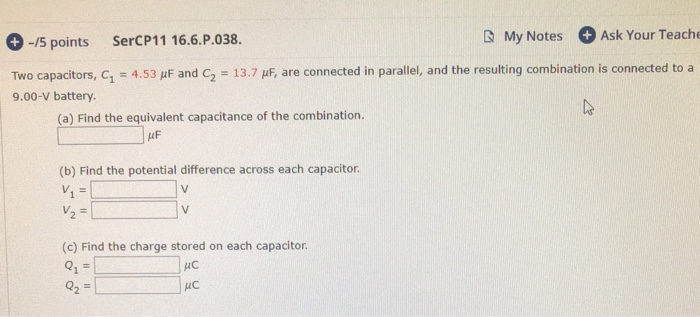+-/5 points SerCP11 16.6.P.038. B My Notes Ask Your Teach Two capacitors, C = 4.53 uF and C2 = 13.7 uF, are connected in parallel, and the resulting combination is connected to a 9.00-V battery (a) Find the equivalent capacitance of the combination. (b) Find the potential difference across each capacitor. V2 = V2 = (c) Find the charge stored on each capacitor. Q= Q2 = uc uc

Free Homework Help App